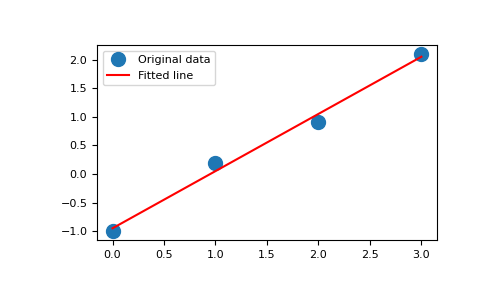# numpy.linalg.lstsq¶

linalg.lstsq(a, b, rcond='warn')[source]

Return the least-squares solution to a linear matrix equation.

Computes the vector x that approximatively solves the equation a @ x = b. The equation may be under-, well-, or over-determined (i.e., the number of linearly independent rows of a can be less than, equal to, or greater than its number of linearly independent columns). If a is square and of full rank, then x (but for round-off error) is the “exact” solution of the equation. Else, x minimizes the Euclidean 2-norm $$||b - ax||$$. If there are multiple minimizing solutions, the one with the smallest 2-norm $$||x||$$ is returned.

Parameters
a(M, N) array_like

“Coefficient” matrix.

b{(M,), (M, K)} array_like

Ordinate or “dependent variable” values. If b is two-dimensional, the least-squares solution is calculated for each of the K columns of b.

rcondfloat, optional

Cut-off ratio for small singular values of a. For the purposes of rank determination, singular values are treated as zero if they are smaller than rcond times the largest singular value of a.

Changed in version 1.14.0: If not set, a FutureWarning is given. The previous default of -1 will use the machine precision as rcond parameter, the new default will use the machine precision times max(M, N). To silence the warning and use the new default, use rcond=None, to keep using the old behavior, use rcond=-1.

Returns
x{(N,), (N, K)} ndarray

Least-squares solution. If b is two-dimensional, the solutions are in the K columns of x.

residuals{(1,), (K,), (0,)} ndarray

Sums of squared residuals: Squared Euclidean 2-norm for each column in b - a @ x. If the rank of a is < N or M <= N, this is an empty array. If b is 1-dimensional, this is a (1,) shape array. Otherwise the shape is (K,).

rankint

Rank of matrix a.

s(min(M, N),) ndarray

Singular values of a.

Raises
LinAlgError

If computation does not converge.

scipy.linalg.lstsq

Similar function in SciPy.

Notes

If b is a matrix, then all array results are returned as matrices.

Examples

Fit a line, y = mx + c, through some noisy data-points:

>>> x = np.array([0, 1, 2, 3])
>>> y = np.array([-1, 0.2, 0.9, 2.1])


By examining the coefficients, we see that the line should have a gradient of roughly 1 and cut the y-axis at, more or less, -1.

We can rewrite the line equation as y = Ap, where A = [[x 1]] and p = [[m], [c]]. Now use lstsq to solve for p:

>>> A = np.vstack([x, np.ones(len(x))]).T
>>> A
array([[ 0.,  1.],
[ 1.,  1.],
[ 2.,  1.],
[ 3.,  1.]])

>>> m, c = np.linalg.lstsq(A, y, rcond=None)
>>> m, c
(1.0 -0.95) # may vary


Plot the data along with the fitted line:

>>> import matplotlib.pyplot as plt
>>> _ = plt.plot(x, y, 'o', label='Original data', markersize=10)
>>> _ = plt.plot(x, m*x + c, 'r', label='Fitted line')
>>> _ = plt.legend()
>>> plt.show()